Test: Radiation Heat Transfer - 1

# Test: Radiation Heat Transfer - 1

Test Description

## 10 Questions MCQ Test Topicwise Question Bank for Mechanical Engineering | Test: Radiation Heat Transfer - 1

Test: Radiation Heat Transfer - 1 for Chemical Engineering 2022 is part of Topicwise Question Bank for Mechanical Engineering preparation. The Test: Radiation Heat Transfer - 1 questions and answers have been prepared according to the Chemical Engineering exam syllabus.The Test: Radiation Heat Transfer - 1 MCQs are made for Chemical Engineering 2022 Exam. Find important definitions, questions, notes, meanings, examples, exercises, MCQs and online tests for Test: Radiation Heat Transfer - 1 below.
Solutions of Test: Radiation Heat Transfer - 1 questions in English are available as part of our Topicwise Question Bank for Mechanical Engineering for Chemical Engineering & Test: Radiation Heat Transfer - 1 solutions in Hindi for Topicwise Question Bank for Mechanical Engineering course. Download more important topics, notes, lectures and mock test series for Chemical Engineering Exam by signing up for free. Attempt Test: Radiation Heat Transfer - 1 | 10 questions in 30 minutes | Mock test for Chemical Engineering preparation | Free important questions MCQ to study Topicwise Question Bank for Mechanical Engineering for Chemical Engineering Exam | Download free PDF with solutions
 1 Crore+ students have signed up on EduRev. Have you?
Test: Radiation Heat Transfer - 1 - Question 1

### The energy emitted (of all wavelengths) in a particular direction per unit surface area and through a unit solid angle is called

Test: Radiation Heat Transfer - 1 - Question 2

### The em issive power is multiplied with the factor ................ to obtain the intensity of normal radiation for a unit surface

Test: Radiation Heat Transfer - 1 - Question 3

### The earth receive at its surface radiation from the sun at the rate of 1400 W/m2. The distance of the centre of sun from the surface of earth is 1.5 x 1011 m and the radius of the sun is 7 x 108 m. Treating the sun as black body, it follows from the above data that sun's surface temperature is about

Detailed Solution for Test: Radiation Heat Transfer - 1 - Question 3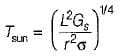where L is mean distance between sun and earth,

r is radius of the sun

Gs is solar constant and equal to 1400 W/m2

σ  is Stefan-Boltzman constan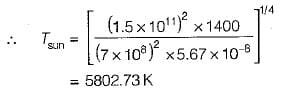Test: Radiation Heat Transfer - 1 - Question 4

What combination of surface characteristics is required for solar collectors?

Test: Radiation Heat Transfer - 1 - Question 5

The radiant heat exchange between two bodies depends upon
1. how they see each other
2. their emitting and absorbing characteristics and
3. the medium that intervenes the two bodies

Which of the above statements are valid?

Test: Radiation Heat Transfer - 1 - Question 6

Consider the following statements pertaining to emissivity:

1. A gray body has variable monochromatic em issivity, ∈λ, with respect to wavelength .
2. A gray body has a characteristic emissivity value of less than unity which does not vary with temperature.
3. According to kirchoff’s law, the emissivity of the surface of a body is equal to its absorptivity when the body is in thermal equilibrium with its surroundings,
4. Kirchoff’s law holds good for monochromatic radiation.

Which of the above statements are valid?

Test: Radiation Heat Transfer - 1 - Question 7

For the same type of shapes , the value of radiation shape factor will be higher when

Test: Radiation Heat Transfer - 1 - Question 8

What is the value of shape factor for two infinite* parallel surfaces separated by a distance x?

Test: Radiation Heat Transfer - 1 - Question 9

What is the view factor F12 for inclined parallel plates of equal width and a common edge?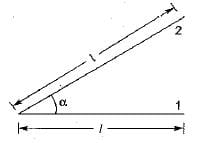Test: Radiation Heat Transfer - 1 - Question 10

What is the view factor F21 for the geometry having one side of a diagonal partition within a Long square duet?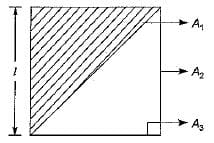Detailed Solution for Test: Radiation Heat Transfer - 1 - Question 10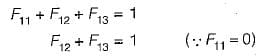By symmetry, F12 = F13 = 0.5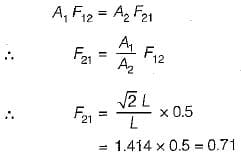## Topicwise Question Bank for Mechanical Engineering

58 videos|314 tests
 Use Code STAYHOME200 and get INR 200 additional OFF Use Coupon Code
Information about Test: Radiation Heat Transfer - 1 Page
In this test you can find the Exam questions for Test: Radiation Heat Transfer - 1 solved & explained in the simplest way possible. Besides giving Questions and answers for Test: Radiation Heat Transfer - 1, EduRev gives you an ample number of Online tests for practice

## Topicwise Question Bank for Mechanical Engineering

58 videos|314 tests